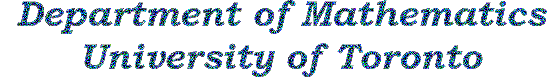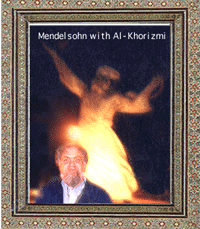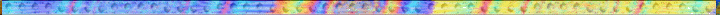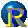combinatorial designs finite geometries graphs codes combinatorial designs finite geometries graphs codes combinatorial designs finite geometries graphs codes combinatorial designs finite geometries graphs codes codes combinatorial designs finite geometries graphs codes combinatorial designs finite geometries graphs codes combinatorial designs finite geometries graphs codes combinatorial designs finite geometries graphs codes combinatorial designs finite geometries graphs codes combinatorial designs finite geometries graphs codes combinatorial designs finite geometries graphs codes combinatorial designs finite geometries graphs codes combinatorial designs finite geometries graphsetries graphs codes combinatorial designs finite geometries graphs codes combinatorial designs finite geometries graphs codes combinatorial designs finite geometries graphs codes codes combinatorial designs finite geometries graphs codes combinatorial designs finite geometries graphs codes combinatorial designs finite geometries graphs codes combinatorial designs finite geometries graphs codes combinatorial designs finite geometries graphs codes combinatorial designs finite geometries graphs codes combinatorial designs finite geometries graphs codes combinatorial designs finite geometries graphs codes combinatorial designs finite geometrie graphs codes combinatorial designs finite geometries graphs codes combinatorial designs finite geometries graphs codes combinatorial designs finite geometries graphs codes combinatorial designs finite geometries graphs codes combinatorial designs finite geometries graphs codes combinatorial designs finite geometries graphs codes combinatorial designs finite geometries graphs codes combinatorial designs finite geometries graphs codes combinatorial designs finite geometries graphs codes combinatorialERIC MENDELSOHNProfessor Emeritus of MathematicsAdjunct Professor of Computer ScienceMendelsohnwithAl  KhorismiTehran 1998

 Department of Mathematics  University of Toronto  Toronto, ON M5S 2E4  Office:Bahen Building , Room 6176  Tel: (416) 978-3462     Fax: (416) 978-4107   email:    mendelso AT     math.utoronto.ca•esearch Interests include
• combinatorial design theory,
• Steiner triple systems
• graph theory,
• software testing arrays,
• finite geometry.